# How to Calculate MAX in Excel

## How to Calculate MAX in Excel

The MAX function in Excel is used to calculate the maximum value within a range of cells.

This tutorial explores how to calculate max values by using the Excel MAX shortcut button, and by writing a MAX formula in the Excel Formula Bar.

Since its introduction in 1985, Microsoft Excel has been a popular tool for people in all walks of life performing a wide variety of calculations. Functions are predefined formulas the perform calculations using specific values in a specific order. Most Excel users will use functions to perform any number of calculations on their worksheets.

The list of Excel functions has grown to over 450 – the good news is that ALL functions follow the same syntax. All functions in Excel follow this syntax =FUNCTION(ARGUMENT). The argument is specifics values or cell references used in the formula.

## How to Calculate MAX using the AutoMAX feature

Popular Excel functions, like MAX, will have one or more shortcut buttons available to let users quickly perform calculations without having to type out the formula in the Excel formula bar.

The AutoMAX feature requires at least one range of numbers and no more than 255.

### Step One: Select the cell where the formula will be placed.

Normally, Excel users will use the AutoMAX feature at the end of a data series in a row or column, but the function can be used in any open cell on the worksheet, as long as the correct cells are referenced.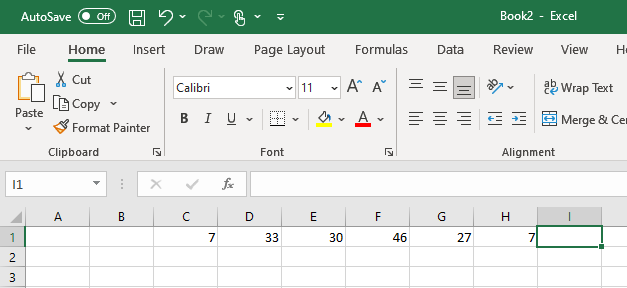### Step Two: Locate the AutoMAX command.

The AutoMAX button can be found in two places in Excel, and characterized by the Greek letter sigma.

The AutoMAX command can be located on the Excel Home Tab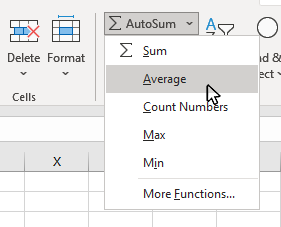or on the Formulas Tab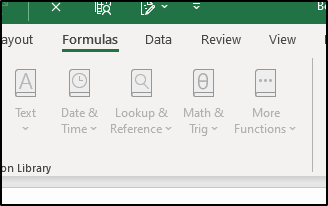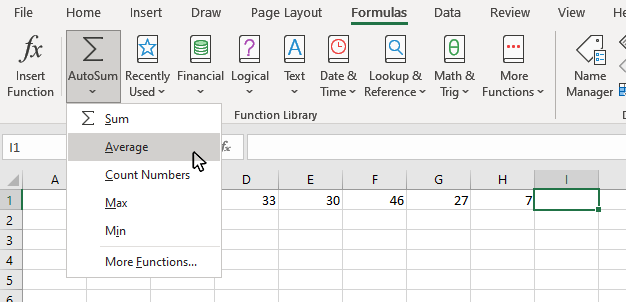### Step Three: Click on the AutoMAX button

Once you click the AutoMAX button you will see the result appear in the cell you originally selected.

Verify the correct range of cells have been highlighted (click and drag with the mouse can be used to change the cells included in the formula).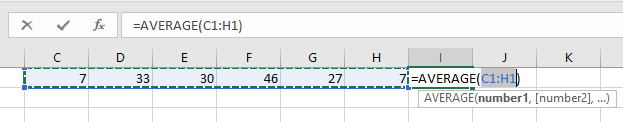### Step Four: Click the Checkmark on the Formula Bar, or click the Enter key

To complete the formula, click the checkmark button on the formula bar, or click the enter key on your keyboard.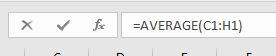### Result: You will see the result of the formula appear in the cell you originally selected.

The cell where you placed the MAX formula will show the MAX of the values in the cell range you selected.

This result is dynamic, and will update automatically if any of the values of the source data changes.## How to Calculate MAX using the MAX Function in Excel

The syntax for the MAX function is =MAX(Number1 : Number2).

The MAX function requires at least one range of numbers and no more than 255.

### Step One: Select the cell where the formula will be placed.

Normally, Excel users will use the MAX function at the end of a data series in a row or column.### Step Two: Type =MAX() in the cell

By typing the equal (=) sign you will initiate a formula in the selected cell. The MAX() function will let Excel know you are performing a MAX calculation.

You would use the same process for any other type of Excel formula.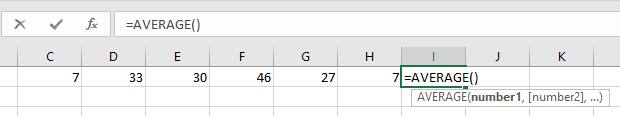### Step Three: Enter the Cell Range inside the MAX formula brackets

You can enter the cell range in the formula by selcting the cell range using your mouse, or by typing the cell references into the formula starting with the first cell in the range and ending with the last.### Step Four: Click the Checkmark on the Formula Bar, or click the Enter key

To complete the formula, click the checkmark button on the formula bar, or click the enter key on your keyboard.### Result: You will see the result of the formula appear in the cell you originally selected.

The cell where you placed the AuotMAX formula will show the MAX of the values in the cell range you selected.

This result is dynamic, and will update automatically if any of the values of the source data changes.Topic #4

Thanks for checking out this tutorial. If you need additional help, you can check out some of our other free Excel Formulas and Functions tutorials, or consider taking an Excel class with one of our professional trainers.